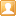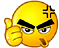##用户名 Email 自动登录 找回密码 密码 注册用户

# 客家文化保护与传承团队，敬请加盟，资源共享，共创未来楼主| 发表于 2008-8-3 17:57:40 | 显示全部楼层
 va=蛙 va=哇 va=娃 va=洼 va=话 vatug=话筒 shuva=树va1（树枝）楼主| 发表于 2008-8-3 17:58:16 | 显示全部楼层
 za=炸 za=诈 za=抓 za=榨 za=吒 za=渣 zang=炸鱼 zariu=榨油 zasi=诈死楼主| 发表于 2008-8-3 17:58:46 | 显示全部楼层
 zha=zha1（伞） zha=遮 zha=者 zha=蔗 zha=鹧 zharam=遮掩 zhachu=遮住 zhabi=遮蔽 zhadog=遮挡 zhagoi=遮盖 zhasiu=遮羞 zhaman=遮瞒 zhalan=遮拦 zhachiu=遮丑 zhagog=遮光 zhatog=蔗糖 zhagu=鹧鸪 zhag=zhag1（正月，一月） zhag=zhag4（刚刚，如“zhag4来－－刚来” zhag=zhag3（修理） zhak=只 zhak=炙 zhakfo=炙火（烤火） zhakse=只数 zham=沾 zham=粘 zham=詹 zham=瞻 zham=占 zhamriu=占有 zhamgi=占据 zhamrug=占用 zhamljag=占领 zhamsjen=占先 zhamti=占地 zhamzhu=占住 zhamsjen=占线 zhambuk=占卜 zhamgwa=占卦 zhamljau=占了 zhamman=占满 zhamgi=占居 zhammi=粘米 zhan=毡 zhan=展 zhan=战 zhanchut=展出 zhanlam=展览 zhankoi=展开 zhanshi=展示 zhanhjen=展现 zhanmog=展望 zhansjau=展销 zhanpin=展品 zhanchigofui=展翅高飞 zhangwan=展馆 zhanfui=展会 zhanvui=展位 zhanbo=战报 zhanchog=战场 zhancjen=战前 zhandeu=战斗 zhanfam=战犯 zhanfo=战火 zhanfu=战俘 zhangam=战舰 zhangi=战机 zhango=战歌 zhango=战果 zhangu=战鼓 zhangug=战功 zhangwet=战国 zhanhau=战壕 zhanheu=战后 zhankjuk=战局 zhankog=战况 zhanli=战例 zhanljok=战略 zhanlon=战乱 zhanma=战马 zhanpai=战败 zhanpi=战备 zhanrit=战役 zhanriu=战友 zhanse=战士 zhanse=战事 zhanshi=战时 zhanshin=战胜 zhansi=战死 zhansjen=战线 zhansut=战术 zhanti=战地 zhanzen=战争 zhanzit=战绩 zhap=折 zhapri=折椅 zhapsan=折伞 zhapshan=折扇 cunzhap=存折 zhat=折 zhat=哲 zhatfuk=折服 zhatga=折价 zhathap=折合 zhatkiu=折旧 zhatkjeu=折扣 zhatmo=折磨 zhatrau=折腰 zhatrog=折让 zhatsat=折杀 zhatsha=折射 zhatshag=折成 zhatson=折算 zhatten=折腾 zhattjap=折叠 zhatton=折断 zhatzhug=折中 zhatzhug=折衷 zhathok=哲学 zhatli=哲理 zhapshan=折扇 zhapri=折椅 zhapsan=折伞 zhau=zhau1（早餐） zhau=朝 zhau=钊 zhau=招 zhau=昭 zhau=召 zhau=诏 zhau=照 zhaushi=朝辰（早上） zhauki=朝气 zhaurog=朝阳 zhausit=朝夕 zhauha=朝霞 zhauban=照搬 zhaucau=照抄 zhauce=照此 zhauchok=照着 zhauden=照登 zhaudo=照到 zhaufu=照付 zhaufui=照会 zhaugu=照顾 zhaugwan=照管 zhaukiu=照旧 zhaukon=照看 zhauli=照理 zhauli=照例 zhauljau=照料 zhauljog=照亮 zhaumin=照明 zhaupan=照办 zhaupjen=照片 zhaurau=照耀 zhaurin=照应 zhaurog=照样 zhausha=照射 zhaushit=照实 zhaushog=照常 zhaushot=照说 zhausjog=照相 zhausjog=照像 zhauzhog=照章 zhaubin=招兵 zhaubjau=招标 zhaucin=招亲 zhaucip=招集 zhaufu=招呼 zhauga=招架 zhaugjug=招供 zhaugu=招股 zhaugug=招工 zhaukau=招考 zhaulai=招徕 zhaulam=招揽 zhauljag=招领 zhauloi=招来 zhaumu=招募 zhaungin=招认 zhaungja=招惹 zhaupai=招牌 zhaupin=招聘 zhaurau=招摇 zhaurin=招引 zhause=招数 zhausen=招生 zhaushit=招式 zhaushiu=招收 zhaushiu=招手 zhaushog=招商 zhautjap=招贴 zhautoi=招待 zhauze=招租 zhauzhan=招展 zhauzhi=招致 zheili=zhei1li2（果冻） zhei=zhei2（用镰刀砍） zhei=zhei3（丑） zhei=这 zheiban=这般 zheibjen=这边 zheibog=这帮 zheibun=这本 zheice=这次 zheichog=这场 zheiciu=这就 zheicoi=这才 zheidjam=这点 zheifan=这番 zheifo=这伙 zheifui=这回 zheifui=这回 zheifuk=这幅 zheifun=这份 zheiga=这家 zheigai=这个 zheigi=这句 zheigu=这股 zheiha=这下 zheihog=这项 zheikan=这款 zheikjen=这件 zheikjun=这群 zheikwai=这块 zheili=这里 zheiljog=这辆 zheilui=这类 zheimo=这么 zheingin=这人 zheipei=这批 zheipu=这部 zheira=这也 zheirau=这要 zheiri=这儿 zheiriu=这又 zheirog=这样 zheise=这使 zheishi=这时 zheishi=这是 zheisja=这些 zheisug=这双 zheitjau=这条 zheitoi=这台 zheiton=这段 zheivui=这位 zheizhak=这只 zheizhog=这张 zheizhug=这种 zheizjog=这将 zhep=zhep1（猪进食的声音） zhet=zhet1（哄骗） letzhet=let1zhet1（很脏） zhi=支 zhi=脂 zhi=止 zhi=只 zhi=旨 zhi=址 zhi=纸 zhi=指 zhi=趾 zhi=志 zhi=制 zhi=挚 zhi=致 zhi=痔 zhi=智 zhi=滞 zhi=痣 zhi=蛭 zhi=置 zhi=膣 zhibai=膣bai2 zhishit=知识 zhicjag=知青 zhingjen=志愿 zhihjog=志向 zhiki=志气 zhici=志趣 zhivan=置换 zhishinsengwai=置身事外 zhingi=置疑 zhiri=置于 zhisin=置信 zhipan=置办 zhingjap=置业 zhihjet=止血 zhitug=止痛 zhichu=止住 zhigin=止境 zhipu=止步 zhikot=止渴 zhikjet=止咳 zhitu=止吐 zhisja=止泻 zhiri=旨意 zhicai=旨在 zhiliu=滞留 zhisjau=滞销 zhizhog=滞胀 zhifog=脂肪 zhifun=脂粉 zhigau=脂膏 zhiriu=挚友 zhioi=挚爱 zhicog=痔疮 zhiba=只把 zhicai=只在 zhidet=只得 zhidog=只当 zhifui=只会 zhigjen=只见 zhigu=只顾 zhigwan=只管 zhihan=只限 zhihei=只系 zhihi=只许 zhiho=只好 zhikiu=只求 zhiko=只可 zhikon=只看 zhimun=只问 zhinen=只能 zhingjen=只愿 zhipa=只怕 zhipin=只凭 zhirau=只要 zhirin=只因 zhiriu=只有 zhirug=只用 zhishi=只是 zhishin=只剩 zhisi=只须 zhisi=只需 zhisjau=只消 zhisjog=只想 zhituk=只读 zhivui=只为 zhizham=只占 zhizjog=只将 zhakshin=只身 zhichok=指着 zhichut=指出 zhidiam=指点 zhifap=指法 zhifui=指挥 zhigap=指甲 zhigau=指教 zhigip=指给 zhihjog=指向 zhikjog=指控 zhilin=指令 zhilu=指路 zhimin=指明 zhimog=指望 zhinamzhim=指南针 zhipai=指派 zhipjau=指标 zhirin=指引 zhise=指使 zhise=指数 zhishi=指示 zhishui=指谁 zhitau=指导 zhiteu=指头 zhitin=指定 zhivun=指纹 zhizhim=指针 zhizhin=指正 zhizit=指责 zhizjam=指尖 zhibjau=制表 zhicai=制裁 zhico=制造 zhiden=制订 zhifuk=制服 zhihen=制衡 zhilen=制冷 zhipin=制品 zhipjen=制片 zhiri=制衣 zhirichog=制衣厂 zhirok=制药 zhirok=制约 zhitin=制定 zhitu=制度 zhitu=制图 zhizhi=制止 zhizok=制作 zhicag=支撑 zhice=支助 zhichi=支持 zhichu=支柱 zhichut=支出 zhici=支取 zhifu=支付 zhigai=支解 zhikan=支款 zhiliu=支流 zhilu=支路 zhingu=支吾 zhipjau=支票 zhipu=支部 zhipui=支配 zhiran=支援 zhitui=支队 zhican=致残 zhice=致词 zhice=致辞 zhicja=致谢 zhifu=致富 zhigin=致敬 zhiham=致函 zhiho=致贺 zhikjap=致歉 zhilit=致力 zhimjag=致命 zhingam=致癌 zhioi=致哀 zhipjag=致病 zhiri=致意 zhirug=致用 zhise=致使 zhishin=致胜 zhishog=致伤 zhisi=致死 zhitjen=致电 zhici=智取 zhifui=智慧 zhikau=智巧 zhili=智利 zhilit=智力 zhiljok=智略 zhimeu=智谋 zhinen=智能 zhinog=智囊 zhinogton=智囊团 zhirug=智勇 zhirugsugcjen=智勇双全 zhiruk=智育 zhishin=智胜 zhishog=智商 zhizha=智者 zhibi=纸币 zhichog=纸厂 zhicjen=纸钱 zhifoi=纸灰 zhigin=纸巾 zhihap=纸盒 zhingjaubu=纸尿布 zhingjaupjen=纸尿片 zhipai=纸牌 zhipjen=纸片 zhisjau=纸屑 zhisjog=纸箱 zhitjau=纸条 zhitoi=纸袋 zhizhog=纸张 zhizjog=纸浆 zhibo=至宝 zhice=至此 zhicin=至亲 zhido=至多 zhigau=至交 zhigauhoriu=至交好友 zhigin=至今 zhigovushog=至高无上 zhigwanchugrau=至关重要 zhiho=至好 zhioi=至爱 zhiri=至于 zhishan=至善 zhishau=至少 zhishin=至诚 zhishog=至上 zhisi=至死 zhitai=至大 zhizun=至尊 zhim=zhim3（扔） zhim=zhim4（蜂刺） zhim=针 zhim=枕 zhimdui=针对 zhimtug=针筒 zhimsjen=针线 zhimgiu=针灸 zhimce=针刺 zhimteu=针头 zhimzhit=针织 zhimfap=针法 zhimfugsjogdui=针锋相对 zhimngan=针眼 zhimgjok=针脚 zhimzjam=针尖 zhimkug=针孔 zhimmuk=枕木 zhimteu=枕头 zhimtau=枕套 zhimgin=枕巾 zhimsit=枕席 zhin=贞 zhin=侦 zhin=珍 zhin=真 zhin=诊 zhin=疹 zhin=振 zhin=赈 zhin=征 zhin=怔 zhin=蒸 zhin=拯 zhin=整 zhin=正 zhin=证 zhin=政 zhin=症 zhinzok=振作 zhinfun=振奋 zhinhin=振兴 zhintug=振动 zhintog=振荡 zhinfuk=振幅 zhinbigofu=振臂高呼 zhincat=侦察 zhintam=侦探 zhinca=侦查 zhincip=侦缉 zhinpo=侦破 zhinvet=侦获 zhinzjet=贞节 zhincau=贞操 zhingjet=贞洁 zhinljet=贞烈 zhinng=贞女 zhinfu=贞妇 zhingwan=贞观 zhinzai=赈灾 zhinzi=赈济 zhincog=症状 zhinheu=症候 zhingjet=症结 zhinng=蒸鱼 zhinlug=蒸笼 zhinfat=蒸发 zhinki=蒸汽 zhinvok=蒸锅 zhinzhinngitshog=蒸蒸日上 zhingiu=拯救 zhinbo=珍宝 zhinchug=珍重 zhincog=珍藏 zhingui=珍贵 zhinhi=珍稀 zhinki=珍奇 zhinkim=珍禽 zhinoi=珍爱 zhinpin=珍品 zhinshi=珍视 zhinsiu=珍馐 zhinsjak=珍惜 zhinzhu=珍珠 zhinbag=真棒 zhinbut=真不 zhinchon=真传 zhincin=真情 zhincjet=真切 zhindi=真知 zhindit=真的 zhinfai=真坏 zhinga=真假 zhingak=真格 zhingam=真敢 zhingim=真金 zhinhjug=真凶 zhinho=真好 zhinkok=真确 zhinkug=真空 zhinkun=真菌 zhinkwai=真快 zhinli=真理 zhinman=真慢 zhinmjag=真名 zhinnen=真能 zhinngin=真人 zhinngjen=真言 zhinngui=真伪 zhinoi=真爱 zhinpi=真皮 zhinpin=真品 zhinri=真意 zhinru=真如 zhinse=真丝 zhinshi=真是 zhinshin=真诚 zhinshin=真身 zhinshit=真实 zhinsim=真心 zhinsjog=真相 zhinti=真谛 zhinva=真话 zhinzhi=真挚 zhinzhin=真正 zhinzhu=真主 zhinchon=震颤 zhingjag=震惊 zhinham=震憾 zhinham=震撼 zhinkip=震级 zhinljet=震裂 zhinngjen=震源 zhinnu=震怒 zhintog=震荡 zhintug=震动 zhincat=诊察 zhinchi=诊治 zhinfui=诊费 zhinljau=诊疗 zhinmak=诊脉 zhinpjag=诊病 zhinshi=诊视 zhinshit=诊室 zhinso=诊所 zhinton=诊断 zhinban=正版 zhinbet=正北 zhinbi=正比 zhinbun=正本 zhinbut=正不 zhincai=正在 zhinchit=正直 zhinchit=正值 zhinchon=正传 zhincon=正餐 zhindjam=正点 zhindog=正当 zhindug=正东 zhindui=正对 zhinfan=正反 zhinfog=正方 zhinfoghin=正方形 zhinfu=正负 zhinfu=正副 zhingin=正经 zhingui=正规 zhingui=正轨 zhinho=正好 zhinkau=正巧 zhinki=正气 zhinkok=正确 zhinlu=正路 zhinmjen=正面 zhinmun=正门 zhinnam=正南 zhinng=正午 zhinngi=正义 zhinngingjunze=正人君子 zhinngjap=正业 zhinpai=正牌 zhinpai=正派 zhinpin=正品 zhinpu=正步 zhinrau=正要 zhinru=正如 zhinse=正事 zhinshi=正视 zhinshi=正是 zhinshit=正式 zhinshog=正常 zhinsi=正西 zhinsjog=正想 zhinti=正题 zhinto=正道 zhintug=正统 zhinvun=正文 zhinzhit=正职 zhinzhug=正中 zhinzug=正宗 zhinban=整版 zhincei=整齐 zhincha=整车 zhinchi=整治 zhindjam=整点 zhindun=整顿 zhingai=整个 zhingi=整机 zhingi=整句 zhingjet=整洁 zhingoi=整改 zhingu=整蛊 zhinhin=整形 zhinkwa=整跨 zhinkwai=整块 zhinli=整理 zhinngin=整人 zhinngit=正日 zhinngjen=整年 zhinpjen=整编 zhinra=整夜 zhinrug=整容 zhinse=整数 zhinsiu=整修 zhintau=整套 zhinti=整体 zhintjen=整天 zhintoi=整台 zhinvan=整晚 zhinzhin=整整 zhinzog=整装 zhinbjen=政变 zhincak=政策 zhinchi=政治 zhindog=政党 zhinfap=政法 zhinfu=政府 zhingai=政界 zhingjen=政见 zhinhjap=政协 zhinkak=政客 zhinkjen=政权 zhinkjuk=政局 zhinshim=政审 zhintam=政坛 zhinti=政体 zhinvu=政务 zhinvui=政委 zhinzjak=政绩 zhince=证词 zhinfap=政法 zhinfun=证婚 zhingi=证据 zhinkjen=证件 zhinkjen=证券 zhinmin=证明 zhinngin=证人 zhinngjen=证言 zhinshit=证实 zhinshu=证书 zhinvut=证物 zhinbin=征兵 zhinchau=征召 zhinchau=征兆 zhinchin=征程 zhincip=征集 zhinden=征订 zhinfuk=征服 zhinfun=征婚 zhingau=征稿 zhingjau=征缴 zhingjeu=征购 zhingwan=征管 zhinkiu=征求 zhinpin=征聘 zhinrug=征用 zhinshiu=征收 zhinshoi=征税 zhinsun=征询 zhintu=征途 zhinvun=征文 zhinzhan=征战 zhip=汁 zhip=执 zhiphag=执行 zhipfap=执法 zhipzhau=执照 zhipri=执意 zhipshu=执输 zhiplip=执笠 zhipzhog=执掌 zhipzhin=执政 zhipkjun=执勤 zhipchok=执着 zhipgau=执教 zhipbit=执笔 zhipau=执拗 zhipbjen=执鞭 zhiprit=汁液 zhit=织 zhit=职 zhit=帜 zhitngjap=职业 zhitvu=职务 zhitgug=职工 zhitzit=职责 zhitran=职员 zhitvui=职位 zhitchin=职称 zhitkjen=职权 zhitnen=职能 zhitshiu=职守 zhitpjet=职别 zhitzhog=职掌 zhitsam=织衫 zhitbu=织布 zhitvut=织物 zhitgi=织机 zhitshin=织成 zhitgug=织工 zhitpin=织品 zhitco=织造 zhiu=周 zhiu=州 zhiu=洲 zhiu=舟 zhiu=昼 zhiu=咒 zhiushi=昼时（上午） zhiura=昼夜 zhiuran=州县 zhiulip=周立 zhiuzhog=州长 zhiuzi=洲际 zhiusan=舟山 zhiushon=舟船 zhiucha=舟车 zhiukjau=舟桥 zhiuze=舟子 zhiuzhug=舟中 zhiungi=咒语 zhiuma=咒骂 zhiubjen=周边 zhiubo=周报 zhiucjen=周全 zhiudi=周知 zhiudo=周到 zhiugug=周公 zhiuhjen=周旋 zhiukan=周刊 zhiuki=周期 zhiuljuk=周六 zhiumat=周末 zhiumet=周密 zhiung=周五 zhiungi=周二 zhiungit=周日 zhiungjen=周年 zhiuri=周易 zhiurit=周一 zhiuriu=周游 zhiusam=周三 zhiusei=周岁 zhiushin=周身 zhiusi=周四 zhiusjog=周详 zhiuvui=周围 zhiuzhat=周折 zhiuzhon=周转 zho=zho2（尿，一般对小孩说） ozho=屙zho2（撒尿） zhog=长 zhog=张 zhog=章 zhog=彰 zhog=漳 zhog=獐 zhog=樟 zhog=璋 zhog=蟑 zhog=涨 zhog=掌 zhog=帐 zhog=胀 zhog=账 zhog=粀 zhog=障 zhog=嶂 zhogpon=粀饭（盛饭） zhogngoi=障碍 zhogbi=障蔽 zhogngan=障眼 zhogzhiu=漳州 zhogshuha=樟树下 zhogno=樟脑 zhogmuk=樟木 zhogshu=樟树 zhoglog=蟑螂 zhogden=掌灯 zhogdo=掌舵 zhoggu=掌故 zhoggwan=掌管 zhogkjen=掌权 zhogkjog=掌控 zhogkui=掌柜 zhogmun=掌门 zhogngjeu=掌牛 zhogshag=掌声 zhogshog=掌上 zhogshogminzhu=掌上明珠   zhogshok=掌勺 zhogsim=掌心 zhogvok=掌握 zhogvun=掌纹 zhogzui=掌嘴 zhoggwa=张挂 zhogkjeu=张口 zhogkoi=张开 zhoglo=张罗 zhogmog=张望 zhogrog=张扬 zhogtai=张大 zhogtjap=张贴 zhogzui=张嘴 zhogbui=长辈 zhoggwan=长官 zhoghjug=长兄 zhogman=长满 zhogsjog=长相 zhogtai=长大 zhogze=长子 zhogzha=长者 zhogchin=章程 zhogfap=章法 zhoggi=章句 zhogng=章鱼 zhogzjet=章节 zhogbun=帐本 zhogcak=帐册 zhogdan=帐单 zhogfog=帐房 zhogfu=帐户 zhogho=帐号 zhogkan=帐款 zhogmjen=帐面 zhogmuk=帐目 zhogpu=帐簿 zhogpug=帐蓬 zhogpug=帐篷 zhogshog=帐上 zhogbun=账本 zhogcak=账册 zhogdan=账单 zhogfog=账房 zhogfu=账户 zhoggi=账据 zhogho=账号 zhogkan=账款 zhogmjen=账面 zhogmuk=账目 zhogpu=账簿 zhogvu=账务 zhogchau=涨潮 zhogdjet=涨跌 zhogfuk=涨幅 zhogga=涨价 zhoglok=涨落 zhogshei=涨势 zhogshui=涨水 zhogtim=涨停 zhoi=zhoi4（嘴巴） zhok=zhok3（穿衣） zhok=斫 zhon=zhon4（倒下） zhon=专 zhon=砖 zhon=转 zhonchog=砖厂 zhonchog=砖场 zhoncjog=砖墙 zhonkwai=砖块 zhonngwa=砖瓦 zhonrau=砖窑 zhontap=砖塔 zhonteu=砖头 zhoncha=专车 zhonchin=专程 zhonchog=专长 zhonfog=专访 zhonga=专家 zhongi=专机 zhongui=专柜 zhongwan=专管 zhonhog=专项 zhonkan=专刊 zhonkan=专款 zhonko=专科 zhonlan=专栏 zhonli=专利 zhonljet=专列 zhonmai=专卖 zhonmun=专门 zhonngin=专人 zhonngjap=专业 zhonran=专员 zhonrit=专一 zhonrug=专用 zhonsim=专心 zhonsjen=专线 zhonti=专题 zhonvag=专横 zhonvui=专为 zhonzhi=专制 zhonzhin=专真（故意） zhonzhin=专政 zhonzhit=专职 zhonzhu=专注 zhonzhu=专著 zhonzit=专责 zhonbjen=转变 zhonbo=转播 zhoncha=转车 zhonchut=转出 zhondo=转到 zhonfa=转化 zhonfat=转发 zhonfui=转回 zhonga=转嫁 zhongau=转交 zhongau=转校 zhongi=转机 zhongip=转给 zhongo=转告 zhongo=转过 zhongok=转角 zhongui=转归 zhongui=转轨 zhonhjag=转型 zhonhjog=转向 zhonhog=转行 zhonhok=转学 zhonki=转去 zhonloi=转来   zhonlu=转而 zhonmai=转卖 zhonngan=转眼 zhonngip=转入 zhonngjam=转念 zhonngjap=转业 zhonri=转移 zhonrog=转让 zhonrug=转用 zhonrun=转运 zhonsan=转产 zhonshei=转世 zhonshi=转成   zhonshin=转身 zhonshiu=转手 zhonshun=转瞬 zhonsug=转送 zhonsut=转述 zhontat=转达 zhonteu=转头 zhonti=转递 zhontug=转动 zhonvan=转换 zhonvan=转弯 zhonvui=转为 zhonvukka=转屋家 zhonzai=转载 zhonze=转租 zhonzen=转赠 zhonzhat=转折 zhonzhi=转制 zhonzhin=转正 zhonzhog=转帐 zhonzhog=转账 zhonzja=转借 zhonzjap=转接 zhu=朱 zhu=诛 zhu=株 zhu=珠 zhu=诸 zhu=猪 zhu=铢 zhu=蛛 zhu=主 zhu=煮 zhu=注 zhu=驻 zhu=著 zhu=蛀 zhu=铸 zhuri=注意 zhuchug=注重 zhucak=注册 zhusha=注射 zhumuk=注目 zhutin=注定 zhugai=注解 zhumin=注明 zhushi=注视 zhungip=注入 zhusit=注释 zhurim=注音 zhusjau=注销 zhuman=注满 zhushui=注水 zhuze=注资 zhuhoi=珠海 zhuze=珠子 zhubo=珠宝 zhugi=珠玑 zhungjuk=珠玉 zhugog=珠江 zhuson=珠算   zhufui=煮沸 zhugun=煮滚 zhushuk=煮熟 zhufan=煮饭 zhura=煮野 zhucoi=煮菜 zhulan=煮烂 zhuzhuk=煮粥 zhuzhau=煮朝 zhuzhiu=煮昼 zhura=煮夜 zhumjen=煮面 zhutog=煮汤 zhushit=煮食 zhushiu=驻守 zhuzat=驻扎 zhuti=驻地 zhugjun=驻军 zhufa=驻华 zhuzjuk=驻足 zhufog=驻防 zhugogputui=驻港部队   zhuco=铸造 zhukjen=铸件 zhutjet=铸铁 zhushin=铸成 zhugog=铸钢 zhubi=铸币 zhushak=铸石 zhuru=诸如 zhudo=诸多 zhugotljog=诸葛亮 zhuvui=诸位 zhugjun=诸君 zhuheu=诸侯 zhufug=朱红 zhusa=朱砂 zhuzjok=朱雀 zhuljen=株连 zhuzhiu=株州 zhumjag=著名 zhuzok=著作 zhuchin=著称 zhushu=著书 zhusut=著述 zhuchug=蛀虫 zhunga=蛀牙 zhuchi=蛀齿 zhuru=侏儒 zhuri=茱萸   zhuban=主板 zhuce=主次 zhuchi=主持 zhuchi=主治 zhuchog=主场 zhuda=主打 zhufam=主犯 zhufu=主妇 zhufun=主婚 zhugau=主教   zhugi=主机 zhugjen=主见 zhugog=主讲 zhugok=主角 zhugon=主干 zhugu=主顾 zhugug=主攻 zhugwan=主观 zhugwan=主管 zhukau=主考 zhukjen=主权 zhulit=主力 zhuliu=主流 zhuljog=主粮 zhumeu=主谋 zhungi=主义 zhungim=主任 zhungin=主人 zhupan=主办 zhupjen=主编 zhuran=主演 zhurau=主要 zhuri=主意 zhusai=主帅 zhushit=主食 zhusit=主席 zhusiu=主修 zhusug=主送 zhutau=主导 zhuti=主题 zhuti=主体 zhutug=主动 zhutui=主队 zhuzai=主宰 zhuzhi=主旨 zhuzhin=主政 zhuzhog=主张 zhuzjog=主将 zhucap=猪杂 zhuchog=猪肠 zhuchog=猪场 zhudeu=猪窦 zhudu=猪肚 zhufug=猪红 zhugjok=猪脚 zhugon=猪肝 zhugug=猪公 zhugut=猪骨 zhugutteu=猪骨头 zhuhjet=猪血 zhukjen=猪圈 zhulo=猪猡 zhulug=猪笼 zhulugngipshui=猪笼入水   zhuma=猪姆 zhumo=猪毛 zhungjuk=猪肉 zhuno=猪脑 zhupa=猪排 zhupi=猪皮 zhupui=猪肺 zhurau=猪腰 zhuriu=猪油 zhushi=猪屎 zhushit=猪食 zhushiu=猪手 zhusim=猪心 zhutei=猪蹄 zhuteu=猪头 zhutui=猪腿 zhuzai=猪崽 zhuzau=猪爪 zhuze=猪仔 zhuzhip=猪汁 zhuzhoi=猪嘴 zhug=zhug1（擂，撞） zhug=zhug4（掉下悬崖） zhug=舂 zhug=中 zhug=忠 zhug=终 zhug=钟 zhug=衷 zhug=肿 zhug=种 zhug=冢 zhug=踵 zhug=仲 zhug=众 zhugco=种造（人品） zhugcoi=种菜 zhuglui=种类 zhugze=种子 zhugchit=种植 zhugcuk=种族 zhugti=种地 zhugfa=种花 zhugco=种造 zhugshu=种树 zhugtjen=种田   zhugngin=众人 zhugdo=众多 zhugmog=众望 zhugshot=众说 zhugsen=众生 zhugmuk=众目 zhugkjeu=众口 zhugngi=众议 zhugvui=众位 zhugnu=众怒 zhugzhishinshag=众志成城    zhugdjam=终点 zhugri=终于 zhuggjet=终结 zhugzhi=终止 zhugngit=终日 zhuggiu=终究 zhugsen=终生 zhugdon=终端 zhugkip=中级 zhugngjen=终年 zhugchog=终场 zhuggui=终归 zhugkjuk=终局 zhugshin=终身 zhugshin=终成 zhugljau=终了 zhugshim=终审   zhugteu=钟头 zhugcin=钟情 zhugshag=钟声 zhugbjau=钟表 zhugri=钟意 zhugdjam=钟点 zhugdjamgug=钟点工 zhugoi=钟爱 zhugleu=钟楼 zhuggu=钟鼓 zhugbai=钟摆   zhugshit=忠实 zhugshin=忠诚 zhugsim=忠心 zhugri=忠于 zhuggo=忠告 zhugngi=忠义 zhugheu=忠厚 zhugchin=忠臣 zhugsin=忠信 zhugljog=忠良 zhugzhin=忠贞 zhugngjen=忠言 zhugngjenngjakngi=忠言逆耳 zhugshun=忠顺 zhughau=忠孝 zhugljet=忠烈 zhugfun=忠魂   zhugsim=衷心 zhugcin=衷情 zhugkjuk=衷曲 zhugchog=衷肠 zhugliu=肿瘤 zhugtai=肿大 zhugkwai=肿块 zhugtug=肿痛 zhugzhog=肿胀 zhugkiu=舂丘 zhugcai=仲裁   zhuga=中亚 zhugba=中巴 zhugban=中班 zhugbjau=中标 zhugcai=中彩 zhugcen=中层 zhugciu=中秋 zhugcon=中餐 zhugden=中等 zhugdjam=中点 zhugdog=中档 zhugdug=中东 zhugfa=中华 zhugfap=中法 zhugfog=中方 zhugfug=中风 zhugfug=中锋 zhugga=中加 zhuggai=中介 zhuggau=中校 zhuggjei=中计 zhuggjen=中坚 zhuggjen=中间 zhuggwet=中国 zhugha=中下 zhughin=中兴 zhughjag=中型 zhugho=中号 zhughog=中行 zhughok=中学 zhughon=中韩 zhugkau=中考 zhugki=中期 zhugkip=中级 zhugkjen=中肯 zhugkjug=中共 zhugkon=中看 zhuglip=中立 zhuglu=中路 zhugmui=中美 zhugng=中午 zhugngit=中日 zhugngjen=中年 zhugngjen=中原 zhugngo=中俄 zhugngwai=中外 zhugpu=中部 zhugpu=中铺 zhugrat=中越 zhugri=中医 zhugri=中意 zhugrin=中英 zhugrog=中央 zhugrok=中药 zhugrug=中庸 zhugrug=中用 zhugsan=中山 zhugse=中苏   zhugshit=中式 zhugshog=中伤 zhugshog=中上 zhugshu=中枢 zhugshu=中暑 zhugsi=中西 zhugsim=中心 zhugsin=中信   zhugsin=中性 zhugsjau=中小 zhugsun=中旬 zhugtai=中大 zhugtan=中弹 zhugten=中听 zhugton=中断 zhugtu=中途 zhugtui=中队 zhugtuk=中毒 zhugvun=中文 zhugzhau=中招 zhugzhi=中止 zhugzhi=中指   zhugzhon=中专 zhugzhon=中转 zhugzjen=中箭 zhugzjog=中奖 zhui=追 zhui=椎 zhui=锥 zhui=坠 zhui=缀 zhui=赘 zhuilok=坠落 zhuifui=坠毁 zhuilok=坠落 zhuigi=坠机 zhuileu=坠楼 zhuiha=坠下   zhuihap=缀合 zhuigut=椎骨 zhuiti=锥体 zhuihin=锥形 zhuingjuk=赘肉   zhuibin=追兵 zhuibu=追补 zhuibug=追捧 zhuica=追查 zhuichuk=追逐 zhuicim=追寻 zhuida=追打 zhuido=追到 zhuifui=追回 zhuifui=追悔 zhuiga=追加 zhuigit=追击 zhuigiu=追究 zhuigjau=追缴 zhuigon=追赶 zhuikan=追款 zhuikiu=追求 zhuimun=追问 zhuingin=追认 zhuipu=追捕 zhuipui=追肥 zhuiri=追忆 zhuisat=追杀 zhuisencuk=追星族 zhuishiu=追授 zhuishog=追上 zhuisok=追溯 zhuisui=追随 zhuitjaufui=追悼会 zhuito=追讨 zhuizjug=追踪 zhuizog=追赃 zhuk=蜀 zhuk=粥 zhuk=竹 zhuk=竺 zhuk=烛 zhuk=嘱 zhuk=瞩 zhuk=祝 zhuk=筑 zhuk=浊 zhuklim=竹林 zhukgon=竹竿 zhukgon=竹杆 zhukze=竹子 zhukban=竹板 zhuklim=竹林 zhukran=竹园 zhuklam=竹篮 zhukki=竹器 zhukrap=竹叶 zhuktug=竹筒 zhuksun=竹笋 zhukgi=竹枝 zhukgo=竹篙   zhukho=祝贺 zhukfuk=祝福 zhukngjen=祝愿 zhukshiu=祝寿 zhukziu=祝酒 zhukce=祝词   zhukgog=烛光 zhuktoi=烛台 zhuklu=筑路 zhukki=筑起 zhukcau=筑巢 zhukti=筑堤 zhukmuk=瞩目 zhukfu=嘱咐 zhuktok=嘱托 zhun=zhun1（发抖） zhun=准 zhun=圳 zhunpi=准备 zhunkok=准确 zhunshi=准时 zhunzet=准则 zhunhi=准许 zhunri=准予 zhunzjog=准将 zhundjam=准点 zhunshin=准绳 zhunsin=准信 zhunchin=准秤 zhunsen=准星 zhunsinlog=准新郎 zhunsinngjog=准新娘 zhunbaba=准爸爸 zhunmama=准妈妈楼主| 发表于 2008-8-3 17:59:10 | 显示全部楼层
 zja=zja4（水落到热东西上的声音） zja=zja1（漂亮） zja=姐 zja=借 zjakjeu=借口 zjace=借助 zjarug=借用 zjakan=借款 zjagam=借鉴 zjatoi=借贷 zjatjau=借条 zjazai=借债 zjagu=借故 zjashu=借书 zjacjen=借钱 zjafog=借方 zjagi=借机 zjagi=借据 zjasjuk=借宿 zjachu=借出 zjato=借道 zjagip=借给 zjagog=借光 zjafo=借货   zjazja=姐姐 zjafu=姐夫 zjamoi=姐妹 zjati=姐弟 zjagug=姐公（外公） zjapo=姐婆（外婆） auzja=au2zja2（逗小孩玩的一种动作，间隔遮住脸） zjag=zjag1（漂亮） zjag=zjag4（倒转） zjag=井 zjagdei=井底 zjagdeizeva=井底之蛙   zjagga=井架 zjagha=井下 zjagkjeu=井口 zjagran=井然 zjagshui=井水 zjak=迹 zjak=zjak1（木材断裂的声音） zjak=zjak1（吃，潮来语） zjaksjog=迹象 zjam=尖 zjamdag=尖顶 zjamdo=尖刀 zjamdon=尖端 zjamgjau=尖叫 zjamkjet=尖刻 zjamli=尖利 zjamrui=尖锐 zjamsei=尖细 zjamsonkjetpok=尖酸刻薄 zjamze=尖子 zjamzesen=尖子生   zjamzui=尖嘴 zjap=接 zjapban=接班 zjapbok=接驳 zjapcha=接车 zjapchok=接着 zjapchu=接住 zjapchuk=接触 zjapci=接取 zjapcja=接洽 zjapdo=接到 zjapfat=接活 zjapfo=接火 zjapfug=接风 zjapgi=接机 zjapgjen=接见 zjapgo=接过 zjapgui=接轨 zjapgwan=接管 zjapha=接下 zjapkak=接客   zjapkiu=接球 zjapkjeu=接口 zjapkjun=接近 zjaplit=接力 zjapljen=接连 zjaploi=接来 zjapnap=接纳 zjapngim=接任 zjapngin=接人 zjapnginljensam=接二连三 zjapngip=接入 zjaprin=接应 zjaprog=接壤 zjapsen=接生 zjapshiu=接收 zjapshiu=接手 zjapshiu=接受 zjapshog=接上 zjapsjen=接线 zjapsug=接送 zjaptei=接替 zjapten=接听 zjapteu=接头 zjaptoi=接待 zjaptug=接通 zjapvun=接吻 zjapzeu=接走 zjapzi=接济 zjapzin=接进 zjau=焦 zjau=浇 zjau=剿 zjau=礁 zjau=椒 zjau=蕉 zjaudjam=焦点 zjaugip=焦急 zjauli=焦虑 zjauki=焦距 zjaucau=焦躁 zjaushok=焦灼 zjauvog=焦黄 zjaukot=焦渴 zjausim=焦心 zjautu=焦土 zjautan=焦炭 zjaumoi=焦煤 zjauriu=焦油 zjauzok=焦作   zjaumet=剿灭 zjauchu=剿除 zjaufui=剿匪   zjaushui=浇水 zjaushak=礁石 zjen=剪 zjen=煎 zjen=箭 zjen=荐 zjen=zjen4（踢） zjenteu=箭头 zjenpu=箭步 zjendo=剪刀 zjencai=剪彩 zjencip=剪辑 zjencai=剪裁 zjenzhi=剪纸 zjenze=剪子 zjenton=剪断 zjentjap=剪贴 zjenchu=剪除 zjenpjau=剪票 zjenbo=剪报 zjentjau=剪掉 zjendon=剪短 zjenzjap=剪接 zjencjet=剪切 zjenki=剪去 zjenha=剪下 zjenrag=剪影 zjenngau=煎熬 zjenrok=煎药 zjenzhu=煎煮 zjenbjag=煎饼 zjendan=煎蛋   zjendui=煎堆 zjet=节 zjetcjen=节前 zjetgam=节减 zjetgangit=节假日 zjetki=节气 zjetkjag=节俭 zjetliu=节流 zjetlut=节律 zjetmuk=节目 zjetnen=节能 zjetngit=节日 zjetoi=节哀 zjetpok=节拍 zjetrok=节约 zjetruk=节欲 zjetsem=节省 zjetshit=节食   zjetshui=节水 zjettjen=节电 zjetzeu=节奏 zjetzhi=节制 zjo=zjo1（拥挤） zjozjogun=zjo4zjo4gun3（热闹） ozjo=屙zjo2（撒尿） zjog=将 zjog=奖 zjog=蒋 zjog=浆 zjog=酱 zjog=桨 zjog=糨 zjogloi=将来 zjograu=将要 zjogkjun=将近 zjogciu=将就 zjoggjun=将军 zjogri=将于 zjogriu=将有 zjogsjog=将相 zjogsai=将帅 zjogljag=将领 zjogfui=将会 zjogcoi=将才 zjoglin=将令 zjogse=将士 zjoggim=奖金 zjogli=奖励 zjogpin=奖品 zjogchin=奖惩 zjogkjen=奖券 zjogshog=奖赏 zjogcog=奖状 zjogbui=奖杯 zjogpai=奖牌 zjogzhog=奖章 zjogfu=浆糊 zjogriu=酱油 zjogngjuk=酱肉 zjogcoi=酱菜 zjok=雀 zjok=爵 zjok=zjok3（自高自大） zjokrok=雀跃 zjokcau=雀巢 zjokban=雀斑 zjokse=爵士 zjug=纵 zjug=踪 zjugfo=zjug3火（煽火） zjugrug=纵容 zjugvag=纵横 zjugtui=纵队 zjugran=纵然 zjugshin=纵身 zjuggwan=纵观 zjugfo=纵火 zjugse=纵使 zjuggwan=宗贯 zjugcin=纵情 zjugchim=纵深 zjuk=足 zjukcai=足彩 zjukdei=足底 zjukgjen=足见 zjukgjeu=足够 zjukha=足下 zjukhjap=足协 zjukkiu=足球 zjukljen=足联   zjukpu=足部 zjukri=足以 zjuktam=足坛 zjukzhidomeu=足智多谋 zjukzjak=足迹 zjukzjukriuri=足足有余发表于 2008-8-4 01:05:00 | 显示全部楼层
 原帖由 tonghe918 于 2008-7-26 14:36 发表哦，这是个人习惯问题，我喜欢把ang等简化为ag,中介音 u 为 w ， i 为 j。这是为了键盘输入考虑，这样自输出的话跟输入法原有的字库，词组区别比较大， 现在，搜狗，紫光的，可以批量导入自定义词库，如果字音也能够自定义，我们客家人完全可以有一套自己简单快捷的拼音输入方式。 下面，我把个人搜集整理的词条发表出来，供各位参考 我以前看到社区有人喜欢用b/d/g代p/t/k韵尾，所以你把ang简化为ag，会令人误会为ak。我建议还是用p/t/k，ang还是坚持用ang。 [ 本帖最后由 hd136302 于 2008-8-4 01:06 编辑 ]发表于 2008-8-4 12:57:45 | 显示全部楼层
 建议楼主用附件上传方式（如word 、pdf）楼主| 发表于 2008-8-4 19:25:20 | 显示全部楼层
 原帖由 level 于 2008-8-4 12:57 发表建议楼主用附件上传方式（如word 、pdf） 用记事本直接复制过来，是应为，还有几个声母组的词组没有搜集整理好，便于以后编辑。楼主| 发表于 2008-8-18 11:12:45 | 显示全部楼层
 看看那位前辈能多多指教，我这个帖子是探索帖子来的，欢迎各位多提意见． [ 本帖最后由 tonghe918 于 2008-10-16 18:26 编辑 ]楼主| 发表于 2008-10-16 18:25:01 | 显示全部楼层
 原帖由 hd136302 于 2008-8-4 01:05 发表 我以前看到社区有人喜欢用b/d/g代p/t/k韵尾，所以你把ang简化为ag，会令人误会为ak。我建议还是用p/t/k，ang还是坚持用ang。 不错，ang 和　ag 的音　不同发表于 2009-2-22 23:46:45 | 显示全部楼层

## 回复 1# tonghe918 的帖子

 看得出，tonghe918好热心。好专业。仔细看哩全部词库。 有部分纯客家词组，已经采用为新版客家输入法介词源。谢谢。 愿与您探讨两点： 1.过多非客家语言，过于偏向于普通话，是否反而失去保护客家母语介初衷？ 2.编码过于复杂，或会不利于输入。【我之前1.0、2.0共4个版本，就系编码过长而实用性差】发表于 2009-2-23 20:31:03 | 显示全部楼层
 吾同地方个客家话系有差别个，客家话拼音输入法，有利客家话统一口音。 也可以搞“客家话口音输入法”。讲去讲转，全部客家人都爱有统一个意见，拿出过多人讲个客家话口音来做。楼主| 发表于 2009-5-29 17:27:06 | 显示全部楼层
 萧萧,谢谢 你的编码是怎样的，能否给我说一下，我们来共同探讨一下，如何？楼主| 发表于 2010-1-2 01:06:12 | 显示全部楼层
 现在工作比较稳定了，希望可以找几个热心人一起来弄一个客家话拼音输入法词库。 对于我们客家话不同地方口音不同的实际情况，我跟很多客家人打过交道，发现直接用客家话聊天是可以做到的，我们的词汇大概有八成以上是相同的，读音有七成以上是一样的，我们来个求同存异。如何，就是先把相同的字词弄出来，有多种读法的，可以当成同义多音字对待楼主| 发表于 2010-2-27 21:23:42 | 显示全部楼层
 我手头有一本《普通户，广州话，客家话，潮州话，对照读音字典》，里面有四个拼读方案， 我有点搞不懂，就我目前搜集的各种方言的读音，好像可以用一种拼读方案全部拼写出来。也许有其他漏洞，请高手指教哦哦发表于 2010-2-28 19:55:40 | 显示全部楼层

## 回复 59# tonghe918 的帖子

 各种方言的拼音方案本来就是由国际统一的一个国际音标演变而来的，只要采用了国际音标，全世界所有人类的声音都可以用这一套音标拼写出来。

 本版积分规则 回帖后跳转到最后一页